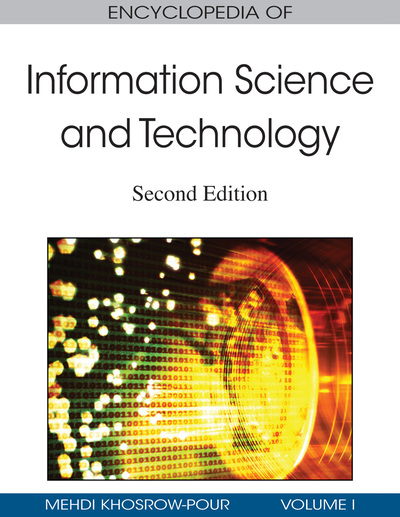# Transforming Recursion to Iteration in Programming

DOI: 10.4018/978-1-60566-026-4.ch603
OnDemand:
(Individual Chapters)
Available
\$37.50
No Current Special Offers

## Abstract

The main advantage of a Recursive Algorithm (an algorithm defined in terms of itself) is that it can be easily described and easily implemented in a programming language (van Breughel, 1997). On the other hand, the efficiency of such an algorithm is relatively low because for every recursive call not yet terminated, a number of data should be maintained in a stack, causing time delays and requiring higher memory space (Rohl, 1984). Solving the same problem iteratively instead of recursively can improve time and space efficiency. For example, to solve a problem that involves N recursive procedure calls, it will require stack space linear to N. On the contrary, using iteration, the program will need a constant amount of space, independent of the number of iterations. There are programming languages, such as Prolog, that do not possess built-in iterative structures and so recursion should be used instead. Nevertheless, there are ways to write recursive programs that have similar behaviour with that of the corresponding iterative programs.
Chapter Preview
Top

## Transforming Recursion To Iteration

The fact is that there is no easy or general way to transform a recursive algorithm to an iterative algorithm. What a programmer can do to increase the efficiency of a recursive algorithm is to implement the recursive algorithm in an iterative manner. This can be done by using special variables called accumulators that will be used to keep intermediate results and facilitate acceleration.

To demonstrate the technique, the calculation of the sum of all integers from 1 to N will be used. The recursive relation that can be used to calculate the sum S of all positive integers from 1 to N is S(N)=S(N-1)+N. A Prolog predicate sum(N,S) that evaluates sum S of all integer numbers from 1 to N, is the following:

```sum(1,1).
sum(N,S):-N1 is N-1,
sum(N1,S1),
S is S1+N.
```

This is a recursive implementation because the second clause involves the call of the same predicate and after it, there is a Prolog system call (S is S1+N). To illustrate the computational effort of the above implementation, the trace of the call to find the sum of all integers from 1 to 4 is shown below (Figure 1).

## Key Terms in this Chapter

Stack Overflow: The condition that occurs when a computer program makes too many calls to subprograms, leading the memory stack to run out of space.

Iteration: The repeated execution of the same process a given number of times or until a specified result is obtained.

TRACE: A report showing the detailed step-by-step execution of a computer program

Predicate: A predicate is an operator or function which returns a Boolean value (e.g., true or false).

Accumulator: A variable used for the accumulation of arithmetic sums, usually evaluated in the form Sum:=Sum+X. Usually, it is initialized with the value zero.

Counter: A variable that stores a single natural number and usually represents the number of iterations that have to be done so far or the number of iterations that are needed from that point on. In the first case, its value is usually evaluated by the form N:=N+1 and its initial value is zero. In the second case, its value is usually evaluated by the form N:=N-1 and its final value is zero.

Recursion: The definition of a program in terms of itself. This gives the program the ability to call itself.

## Complete Chapter List

Search this Book:
Reset